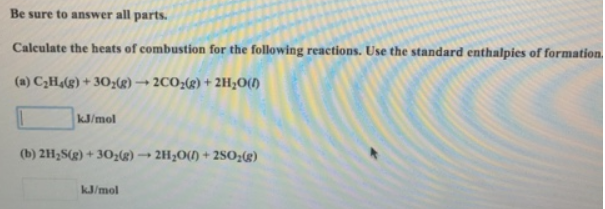# Problem: Be sure to answer all parts.Calculate the heats of combustion for the following reactions. Use the standard enthalpies of formation. (a) C2H4 (g) + 3O2 (g) → 2CO2 (g) + 2H2O (l) kJ/mol (b) 2H2S (g) + 3O2 (g) → 2H2O (l) + 2SO2 (g) kJ/mol

###### FREE Expert Solution
85% (491 ratings)###### Problem Details

Be sure to answer all parts.

Calculate the heats of combustion for the following reactions. Use the standard enthalpies of formation.

(a) C2H4 (g) + 3O2 (g) → 2CO2 (g) + 2H2O (l) kJ/mol

(b) 2H2S (g) + 3O2 (g) → 2H2O (l) + 2SO2 (g) kJ/mol